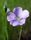## Create macro loop with parameter lists of different lengths

Hello,

I am trying to write a macro loop that can take the following input datasets:

casuser.scored_DT_fold_eq_1_mtd1
casuser.scored_DT_fold_eq_2_mtd1
casuser.scored_DT_fold_eq_3_mtd1
casuser.scored_RF_fold_eq_1_mtd1
casuser.scored_RF_fold_eq_2_mtd1
casuser.scored_RF_fold_eq_3_mtd1

and output the following datasets:

casuser.DT_fold_eq_1_rocinfo_mtd1
casuser.DT_fold_eq_2_rocinfo_mtd1
casuser.DT_fold_eq_3_rocinfo_mtd1
casuser.RF_fold_eq_1_rocinfo_mtd1
casuser.RF_fold_eq_2_rocinfo_mtd1
casuser.RF_fold_eq_3_rocinfo_mtd1

This is the code I have tried so far, with no luck:

``````%macro train(method=,prefix=,name=,fold=);
%do m=1 %to %sysfunc(countw(&method));
%let method=%scan(&method,&m,'~');
%do p=1 %to %sysfunc(countw(&prefix));
%let prefix=%scan(&prefix,&p);
%do n=1 %to %sysfunc(countw(&name));
%let name=%scan(&name,&n);
%do f=1 %to %sysfunc(countw(&fold));
%let fold=%scan(&fold,&f,);
proc assess data=casuser.scored_&prefix._fold_eq_&fold._&method (where=(outer_k_fold ne 1))
ROCOUT=casuser.&prefix._fold_ne_&fold._rocinfo_&method;
input P_target1;
target target / level=nominal event='1';
fitstat pvar=P_target0 / pevent='0';
run;
run;
%end;
%end;
%end;
%end;
%mend train;

%train(method=mtd1, prefix=DT RF, name="Decision_Tree" "Random Forest",fold=1 2 3);``````

I think the errors I am getting have something to do with how the list is written for every macro parameter in the last `%train` statement. Note that the prefix and name macro parameters have lists of the same lengths.

1 ACCEPTED SOLUTION

Accepted Solutions

## Re: Create macro loop with parameter lists of different lengths

You cannot replace the list with a single item and expect it to work for more than one item.

If NAME and PREFIX go together then you only need one %DO loop to cover both lists.

You have to use the same delimiter when counting how many items are in the list as you use when scanning the list.  What is the delimiter for the other lists?  Be specific when telling COUNT() and %SCAN() what delimiter to use.

Are you really using both single quote and ~ as delimiters in the METHOD list? Why?  You appear to be using the value scanned from METHOD as part of a dataset name, so why not just use space as the delimiter?  You can add the Q modifier when scanning the quoted name strings.

Try something like this:

``````%macro train(method=,prefix=,name=,fold=);
%local m p n f nm np nf;
%do nm=1 %to %sysfunc(countw(&method,%str( )));
%let m=%scan(&method,&nm,%str( ));
%do np=1 %to %sysfunc(countw(&prefix,%str( )));
%let p=%scan(&prefix,&np,%str( ));
%let n=%scan(&name,&np,%str( ),q);
%do nf=1 %to %sysfunc(countw(&fold,%str( )));
%let f=%scan(&fold,&nf,%str( ));
%put &=nm &=m &=np &=p &=n &=nf &=f;
proc assess
data=casuser.scored_&p._fold_eq_&f._&m. (where=(outer_k_fold ne 1))
ROCOUT=casuser.&p._fold_ne_&f._rocinfo_&m.
;
input P_target1;
target target / level=nominal event='1';
fitstat pvar=P_target0 / pevent='0';
run;
%end;
%end;
%end;
%mend train;

%train(method=mtd1, prefix=DT RF, name="Decision_Tree" "Random Forest",fold=1 2 3);``````

If I remove the actual SAS code so I can test the macro without your dataset I get:

```554  %train(method=mtd1, prefix=DT RF, name="Decision_Tree" "Random Forest",fold=1 2 3);
NM=1 M=mtd1 NP=1 P=DT N="Decision_Tree" NF=1 F=1
NM=1 M=mtd1 NP=1 P=DT N="Decision_Tree" NF=2 F=2
NM=1 M=mtd1 NP=1 P=DT N="Decision_Tree" NF=3 F=3
NM=1 M=mtd1 NP=2 P=RF N="Random Forest" NF=1 F=1
NM=1 M=mtd1 NP=2 P=RF N="Random Forest" NF=2 F=2
NM=1 M=mtd1 NP=2 P=RF N="Random Forest" NF=3 F=3
```
3 REPLIES 3LinusH
Tourmaline | Level 20

## Re: Create macro loop with parameter lists of different lengths

Analyze (and share) your log with options SYMBOLGEN and MPRINT.

Data never sleeps

## Re: Create macro loop with parameter lists of different lengths

Here's a set of issues you need to attend to.  There could be more of course.

You call the macro with two parameters &NAME and &FOLD which take on multiple values.  But in the middle of executing the macro, you replace &NAME and &FOLD with just a single item from the original list.  You will never be able to go back and retrieve the original lists of values provided when calling the macro.

Instead of replacing &NAME and &FOLD, assign "single item from a list" to a new macro variable.

As a general rule, when you get an error, tell us what the error is.  Better yet, post the log.

## Re: Create macro loop with parameter lists of different lengths

You cannot replace the list with a single item and expect it to work for more than one item.

If NAME and PREFIX go together then you only need one %DO loop to cover both lists.

You have to use the same delimiter when counting how many items are in the list as you use when scanning the list.  What is the delimiter for the other lists?  Be specific when telling COUNT() and %SCAN() what delimiter to use.

Are you really using both single quote and ~ as delimiters in the METHOD list? Why?  You appear to be using the value scanned from METHOD as part of a dataset name, so why not just use space as the delimiter?  You can add the Q modifier when scanning the quoted name strings.

Try something like this:

``````%macro train(method=,prefix=,name=,fold=);
%local m p n f nm np nf;
%do nm=1 %to %sysfunc(countw(&method,%str( )));
%let m=%scan(&method,&nm,%str( ));
%do np=1 %to %sysfunc(countw(&prefix,%str( )));
%let p=%scan(&prefix,&np,%str( ));
%let n=%scan(&name,&np,%str( ),q);
%do nf=1 %to %sysfunc(countw(&fold,%str( )));
%let f=%scan(&fold,&nf,%str( ));
%put &=nm &=m &=np &=p &=n &=nf &=f;
proc assess
data=casuser.scored_&p._fold_eq_&f._&m. (where=(outer_k_fold ne 1))
ROCOUT=casuser.&p._fold_ne_&f._rocinfo_&m.
;
input P_target1;
target target / level=nominal event='1';
fitstat pvar=P_target0 / pevent='0';
run;
%end;
%end;
%end;
%mend train;

%train(method=mtd1, prefix=DT RF, name="Decision_Tree" "Random Forest",fold=1 2 3);``````

If I remove the actual SAS code so I can test the macro without your dataset I get:

```554  %train(method=mtd1, prefix=DT RF, name="Decision_Tree" "Random Forest",fold=1 2 3);
NM=1 M=mtd1 NP=1 P=DT N="Decision_Tree" NF=1 F=1
NM=1 M=mtd1 NP=1 P=DT N="Decision_Tree" NF=2 F=2
NM=1 M=mtd1 NP=1 P=DT N="Decision_Tree" NF=3 F=3
NM=1 M=mtd1 NP=2 P=RF N="Random Forest" NF=1 F=1
NM=1 M=mtd1 NP=2 P=RF N="Random Forest" NF=2 F=2
NM=1 M=mtd1 NP=2 P=RF N="Random Forest" NF=3 F=3
```
Discussion stats
• 3 replies
• 149 views
• 1 like
• 4 in conversation